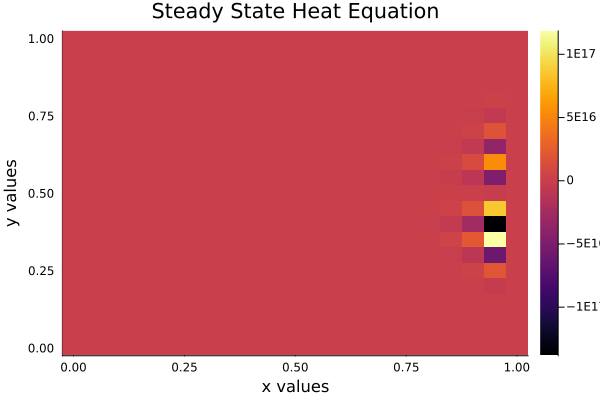# MethodOfLines - numerical instability for example in docs

I am using the steady state heat equation example in the MethodOfLines.jl package.

When I reduce the step side below 0.1 I have a numerical blow-up of the solution.

I do not know if this is only on my end or other users have also faced this error.
To me I do not see a reason for numerical instability of this case. I have attached my code below:

``````using ModelingToolkit, MethodOfLines, DomainSets, NonlinearSolve

@parameters x y
@variables u(..)
Dxx = Differential(x)^2
Dyy = Differential(y)^2

eq = Dxx(u(x, y)) + Dyy(u(x, y)) ~ 0

bcs = [u(0, y) ~ x * y,
u(1, y) ~ x * y,
u(x, 0) ~ x * y,
u(x, 1) ~ x * y]

# Space and time domains
domains = [x ∈ Interval(0.0, 1.0),
y ∈ Interval(0.0, 1.0)]

@named pdesys = PDESystem([eq], bcs, domains, [x, y], [u(x, y)])

dx = 0.05
dy = 0.05

# Note that we pass in `nothing` for the time variable `t` here since we
# are creating a stationary problem without a dependence on time, only space.
discretization = MOLFiniteDifference([x => dx, y => dy], nothing, approx_order=2)

prob = discretize(pdesys, discretization)
sol = NonlinearSolve.solve(prob, NewtonRaphson())

u_sol = sol[u(x, y)]

using Plots

heatmap(sol[x], sol[y], u_sol, xlabel="x values", ylabel="y values",